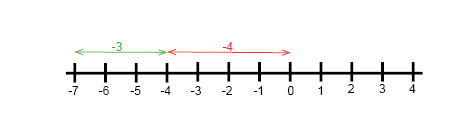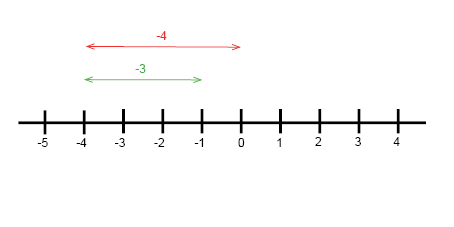You already know how to add 3 + 4 and so on. But there are many ways to add integers. One way to add integers is by using a number line.

Example

$-4+(-3)=\, ?$We always start at zero. Our first number is negative four (-4) so we move 4 units to the left. We then have plus negative three (-3) which is the same as subtracting 3 so we move 3 more units to the left. This gives us the value of negative seven, (-7).

$-4+(-3)=\, ?$

$-4-3=-7$

We do the same thing if we have a positive integer, but instead we move to the right.

$-4+3=\, ?$

$-4+3=-1$You can also add integers and variables.

Example

$13x+(-2)x=\, ?$

$13x-2x=$

$(13-2)x=11x$

When subtracting something from something we wish to find out the difference between the two numbers. When you subtract a negative number from any number the difference is even bigger. The distance from the seabed at a depth of 150ft and an airplane flying at 3000ft altitude at sea level is

$3000-(-150) = 3000 + 150 = 3150ft.$

Thus when we subtract negative numbers, we get:

$4-(-3) = 4 + 3 = 7$

Subtracting −3 is the same as adding 3.

If we have a plus sign before the parentheses then we will not change the signs within the parentheses

If we have a minus sign before the parentheses then we the signs within the parentheses will change.

Two negatives make one positive!

## Video lesson

Calculate -3 + (-6) on a number line